# Deriving the Bond Pricing Formula

Want to know where the bond pricing equation comes from?

Bonds are one of the most common fixed-income investments, and a solid foundation for all investment portfolios.

Many software tools, including Excel, offer functions to compute the value of a bond.

But knowing how the equations are derived gives you far better insight into what the results actually mean.

A bond is a financial instrument in which the bond issuer owes the bond holder

• a periodic payment (known as a coupon, usually paid once or twice a year)
• and the face value (or par value) of the bond, paid at maturity.

Consider a 10-year bond with

• a face value of \$1000
• a coupon rate of 10% (i.e. a yearly coupon payment of \$100)
• and one payment a year

The cash flow paid to the bond-purchaser is illustrated below.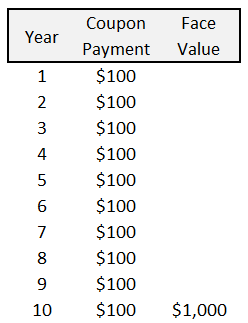The value of a bond is simply the sum of the present value of all the coupon payments and the present value of the face value.

We will now derive the equations used to calculate the value of a bond.

## Present Value of the Coupon Payments

If the coupon payment is C and the interest rate is i, then the present value of the first coupon payment is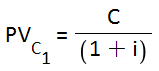The present value of the second coupon payment is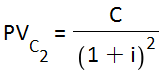If the time to maturity is n, the present value of the final (i.e. nth) coupon payment is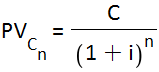Adding together the present value of all coupon payments gives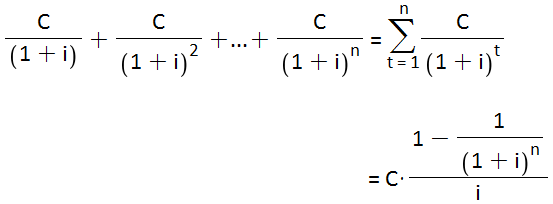## Present Value of the Par Value

The present value of the par value M of the bond (to be paid in period n) is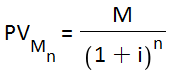## Bond Pricing Equation

The value of the bond is simply the sum of

• the total present value of all coupon payments
• and the present value of par value of the bond

Combining the equations derived above gives.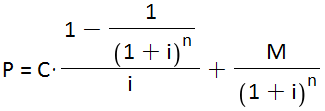This is the actual formula used by Excel’s PV function.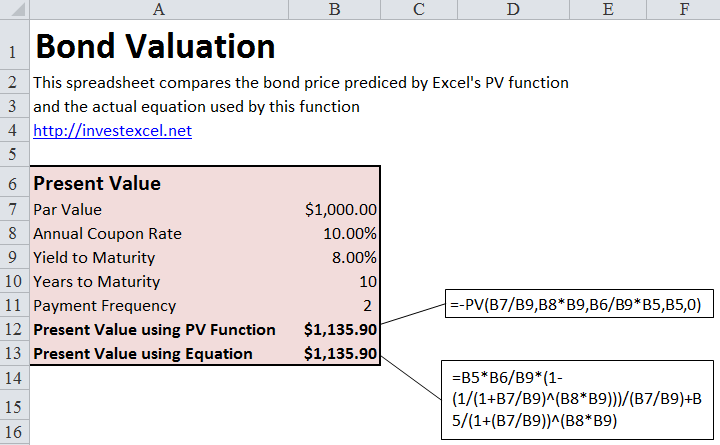## Effect of Coupon Payment Frequency

If there are F coupon payments in every time period (i.e. the payment frequency is F), then

• the coupon payment is divided by F
• the interest rate is divided by F
• the number of payments is multiplied by F

The bond pricing formula then becomes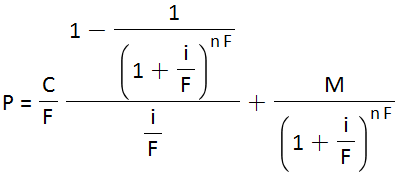As the payment frequency F increases, the bond value increases.

This formula can be rearranged to give the number of payments n.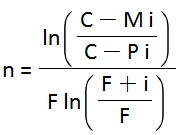The bond pricing equation cannot be rearranged to give an explicit equation for the interest rate. Numerical techniques are required to backsolve the equation for the yield to maturity, given the coupon, face value and payment frequency.

Compare Bond Prices – Excel’s PV function vs Explicit Equation